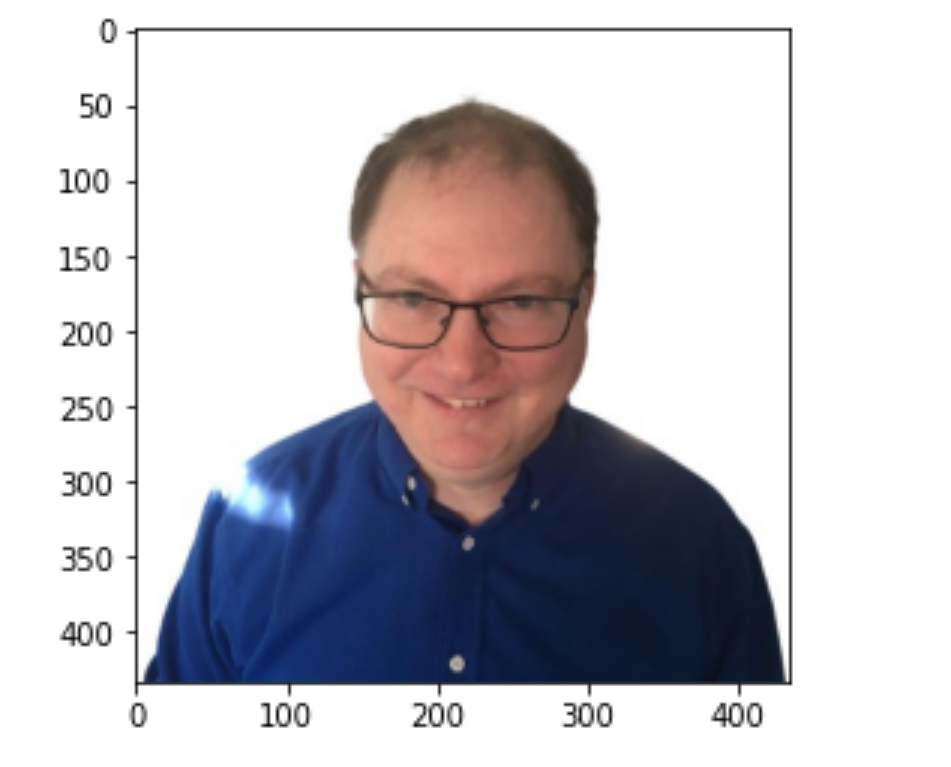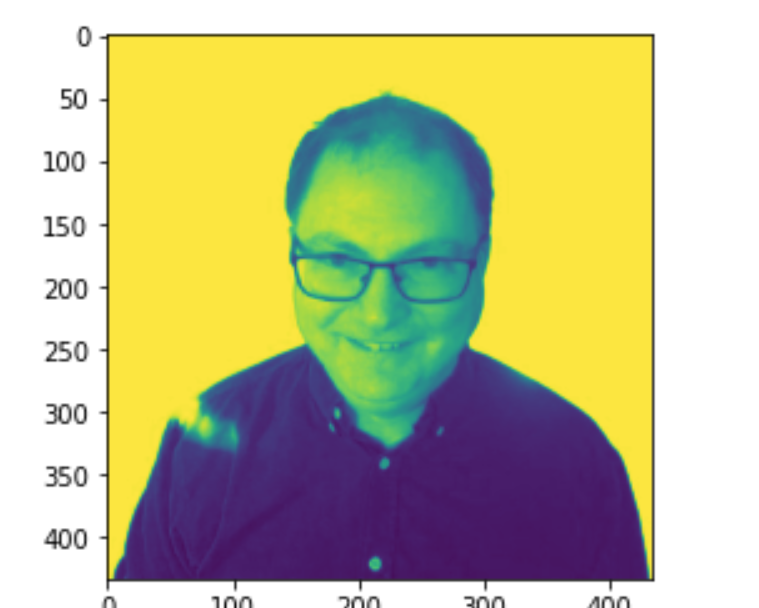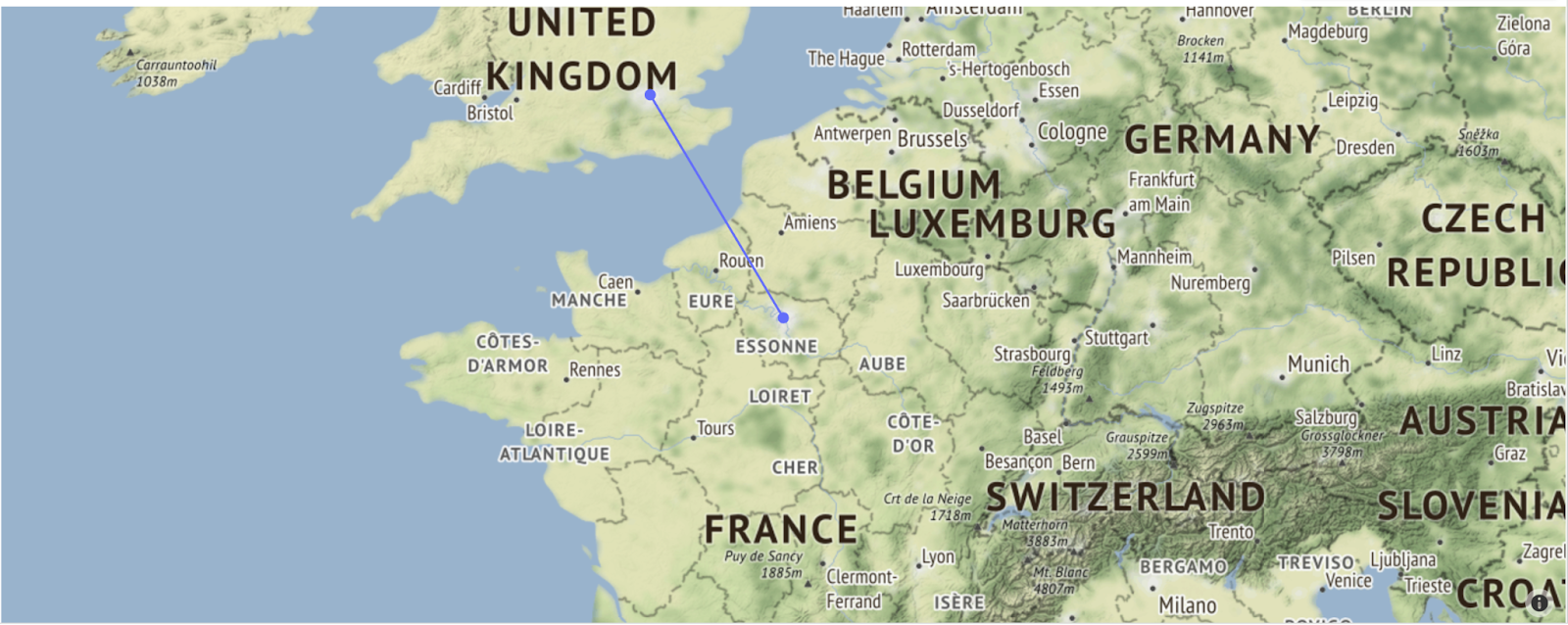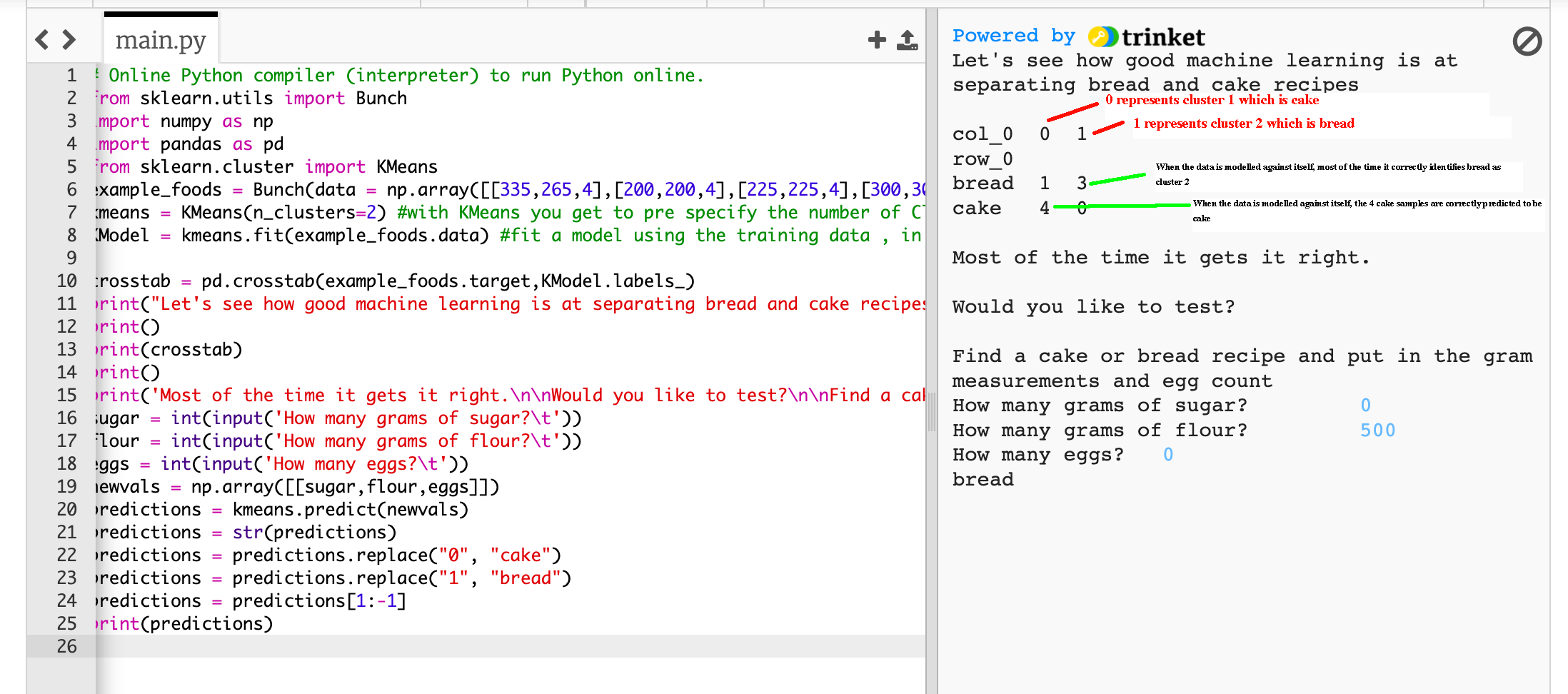# 10 examples of using Python for big data analysis

#### Exploring some of the most powerful Python modules for data analysis###### Christopher Collins

15 minute read · April 11, 2022, 11:30 PM

If you’ve been searching for a job or working in big data, you will know that companies are increasingly expecting you to have experience in Power Bi, Tableau, SQL, MongoDB, and Python, as well as Microsoft Excel. But which one do you learn first to become more valuable in the marketplace?

Learning Python is the logical first step. It can enhance what you can do with all of the above tools and will go a long way in improving your skills and career opportunities in analyzing big data. If you know Python scripting well enough to produce any kind of result or visualization, it may even negate your need to know software packages such as Tableau and Power Bi, which are limited in scope by user interfaces. Python libraries like pandas can also query data to get the same results as SQL can, which is just another reason to consider learning how to program in Python first.

Another benefit of learning Python is that most data-analytics applications have APIs that can be accessed by Python. Beginning with Python gives you potential to be a more advanced user of other data-analytics software. Here are a few specific examples:

• Tableau has an API that can be queried using Python.
• Power Bi supports running Python scripts.
• Python libraries can work with Excel data and automate many Excel functions.
• Python libraries can be used to do SQL queries on structured data sets.
• Python libraries can be used to do NoSQL queries on unstructured data sets.

In this roundup, you’ll explore a few basic code snippets from some core Python analytics libraries that will demonstrate how easy Python is to learn. The time you spend learning Python libraries for data analysis will be a good investment, and its capabilities are being expanded all the time.

The Python libraries that this roundup will review include pandas, NumPy, Matplotlib, Plotly, SciPy, and scikit-learn. These particular libraries have been chosen because they make up the core Python libraries used by data analysts and data scientists. They’re all useful tools that are worth exploring, and you’ll get to see a summary of ten interesting sample projects that leverage Python for big data analysis.

Let’s get started!

## pandas Library for Importing and Finding Data

Reading data is the first step in any data science project. `pandas` is arguably the best Python library to learn about for importing data into any Python analytics project. It’s included in parts of Higher National Diploma analytics courses covering Python as well as MSc in Data Analytics courses.

Data is imported in a pandas DataFrame (df), which can be considered a bit like a database table. The DataFrame can be further queried and manipulated for big data analysis projects. The `pandas` library supports commands that are very similar to SQL for filtering information and can do join analysis the same way SQL does.

### Example 1: Reading Data with pandas

You can use the `pandas` library to import data into a DataFrame from just about any file type, as demonstrated by the code snippet below, which has been adapted from this article.

``````# import pandas library abbreviated as pd
import pandas as pd

# create variable to hold dataframe called df and read the csv file into it

# print result to the screen
print(df)``````

This simple piece of code can tell you a lot about how easy it is to learn Python:

• In the first line of code, Python is importing the pandas library and referencing it as `pd` just so you don’t have to write “pandas” out in full every time you want to use one of its functions.
• The second line of code defines the DataFrame variable as `df` and imports the contents of the csv file into it by using the pandas function `pd.read_csv('file_name.csv')` from the pandas library and applying it to call in the csv file.
• The final line of code prints the contents of the DataFrame to the screen.

Knowing all the functions available for reading different file types is the cornerstone to working with big data. You can also import data in JSON format from online APIs into a DataFrame.

If you are interested in doing so, the code below, adapted from this article, will show you how:

``````import json
import requests
import pandas as pd

# you will need to get a free API key from currencylayer.com

# the requests method is used to get the API url that returns JSON data
r = requests.get("http://api.currencylayer.com/live?access_key=" + apikey +"")

# the json text data is extracted from the url

# the json text data is imported into a pandas dataframe, “quotes” refers to part of the json dictionary
df = pd.DataFrame(jsondata["quotes"], index=)

# with this particular dataset the dataframe(df) needs to be transposed, re indexed, and new column names assigned.
df1_transposed = df.T
df1_transposed = df1_transposed.reset_index()
df1_transposed.columns = ['currency_pair', 'rate']

# view the data extracted from the API
print(df1_transposed)``````

### Example 2: Filtering Data with pandas

You can also use pandas to do SQL-type queries on large data sets. A typical SQL query might look like this:

``````SELECT *
FROM table
WHERE column_name = some_value``````

The same command in `pandas` would look like this:

``df.loc[df['column_name'] == some_value]``

If you are unsure of what analytics technology to learn first and have limited time, you don’t necessarily need to learn SQL before learning Python. All core SQL queries can be done with pandas commands. In time, if you do learn SQL, there are Python libraries such as sqlite3 that provide an interface between Python and SQL syntax.

## NumPy Library for Math Functions and Setting Data Dimensions

Some core uses of the `NumPy` library involve many math functions. There is some overlap between what the `NumPy` library offers and what is in Python’s own math library as the data science and analytics community have widely adopted importing NumPy into Python.

`NumPy` is continuously optimized to work with the latest CPU architectures. Its arrays can work fifty times faster than using Python lists to store data. For that reason, NumPy is frequently used in Python data-science projects, where speed and resources are very important.

### Example 3: Using NumPy Math Functions to Create a Haversine Distance Calculation

`NumPy` supports radians, sin, cos, square root, delta, lambda, arctan, and many more mathematical functions, which can then be combined to make even greater mathematical functions to fit your purpose.

In the example below, a programmer has created a function that calculates Haversine distance. Here, the variables for the longitude and latitude of London and Paris are passed through the function, and the distance is printed on the screen:

``````import numpy as np

def  haversine_distance(lat1, lon1, lat2, lon2):
r = 6371
a = np.sin(delta_phi / 2)**2 + np.cos(phi1) * np.cos(phi2) *  np.sin(delta_lambda / 2)**2
res = r * (2 * np.arctan2(np.sqrt(a), np.sqrt(1 - a)))
return np.round(res, 2)

LondonLongitude = -0.118092
LondonLatitude = 51.509865
ParisLongitude = 2.349014
ParisLatitude = 48.864716

print(haversine_distance(LondonLatitude, LondonLongitude, ParisLatitude, ParisLongitude))``````

### Example 4: Resetting the Dimensions of Data Using NumPy

You can also use `NumPy` to reset the dimensions of data sets extracted from existing DataFrames/lists (e.g., taking a list of eight numbers and converting it into an array of two rows and four columns).

Resetting the dimensions of data is particularly useful for machine learning exercises, where the dimensions of the training data need to match the dimensions of the test data.

``````# importing library
import numpy as np

# initializing list
lst = [1, 7, 0, 5, 6, 2, 5, 6]

# converting list to array
arr = np.array(lst)

# reshape array to 2 rows by four columns
arr = np.reshape(arr,(2,4))

print(arr)``````

For image-recognition scripts, you might have to use `NumPy` to convert the dimensions of an imported image file or files.

## Matplotlib Library for Graph Visualizations and Editing Image Colors

If you’re used to using Excel, Tableau, or PowerBi to create your visualizations, you understand that you can only be as creative with the visualization of your data as the scope of the software allows you to.

The Matplotlib library has a wide range of graphs that cover practically every kind of business use. Check out the template visualizations available on the Matplotlib website gallery page, and adapt online tutorials to meet your data set needs.

Python is a very cohesive programming language, and these visualization libraries often have many tutorials available that can take you step-by-step through how to efficiently work with data imported into either `pandas` DataFrames or `NumPy` data arrays. Let’s take a look at an example of each.

### Example 5: Using Matplotlib to Create a Scatter and Bar Graph

This example shows how to use the Matplotlib library to interpret data from pandas DataFrames into visualizations and graphs. In the code block below, adapted from this article, the data in the DataFrame is manually typed. However, the data could just as easily be imported from a spreadsheet or from an online API.

``````import pandas as pd
import matplotlib.pyplot as plt
df = pd.DataFrame({
'name':['john','mary','peter','jeff','bill','lisa','jose'],
'age':[23,78,22,19,45,33,20],
'gender':['M','F','M','M','M','F','M'],
'state':['california','dc','california','dc','california','texas','texas'],
'num_children':[2,0,0,3,2,1,4],
'num_pets':[5,1,0,5,2,2,3]
})

print(df)

# a scatter plot comparing num_children and num_pets
df.plot(kind='scatter',x='num_children',y='num_pets',color='red')
plt.show()

# a simple bar plot showing the name and age of child
df.plot(kind='bar',x='name',y='age')
plt.show()
``````

### Example 6: Using Matplotlib to Import Image Data as a NumPy Array

More and more training sets in big data use and compare images.

Matplotlib is typically considered to be a library that does graphs, but it can be equally useful for importing images into presentations that you want to create using Python.

``````import matplotlib.pyplot as plt
import matplotlib.image as mpimg
imgplot = plt.imshow(img)
plt.show()
``````Matplotlib can also be used to load images as number arrays that can be used with NumPy:

``````import matplotlib.pyplot as plt
import matplotlib.image as mpimg
print(img)``````

### Example 7: Using Matplotlib to Distort an Image Using NumPy Array Slicing

The values of the numbers that make up an image can be changed or sliced to distort the images, creating more training images from a single image. This is useful as it allows you to create larger training data sets for machine learning image-recognition projects from fewer images.

In the example below, adapted from this tutorial, the image imported using the Matplotlib library is distorted to a different hue:

``````import matplotlib.pyplot as plt
import matplotlib.image as mpimg

# This is array slicing. You can read more in the Numpy tutorial
# https://numpy.org/doc/stable/user/quickstart.html
lum_img = img[:, :, 0]
plt.imshow(lum_img)
``````## Plotly Library for Creating Maps and Graphs

Plotly is another impressive library for making high-quality graphs. Whether you decide to use Matplotlib or Plotly for creating graphs is a matter of personal preference as the syntax is very similar. One important distinction that may give Plotly a leg up, though, is its very impressive gallery of map templates for working with longitude and latitude data.

For a full list of Plotly’s interactive graphs, check out their gallery.

### Example 8: Using Plotly to Plot a Line on a Map from London to Paris

Plotly is excellently suited for big data projects involving map visualizations. Below is some starter code, adapted from this template, to create a map image and draw a line between two longitude and latitude coordinates:

``````import plotly.graph_objects as go

LondonLongitude = -0.118092
LondonLatitude = 51.509865
ParisLongitude = 2.349014
ParisLatitude = 48.864716

fig = go.Figure(go.Scattermapbox(
mode = "markers+lines",
lon = [LondonLongitude, ParisLongitude],
lat = [LondonLatitude, ParisLatitude],
marker = {'size': 10}))
fig.update_layout(
margin ={'l':0,'t':0,'b':0,'r':0},
mapbox = {
'center': {'lon': LondonLongitude, 'lat': LondonLatitude},
'style': "stamen-terrain",
'center': {'lon': ParisLongitude, 'lat': ParisLatitude},
'zoom': 5})
fig.show()
``````## SciPy Library for Regression and Hypothesis Testing

If you haven’t found the mathematical function that you need in Python’s own math library or in NumPy, SciPy is the next library to check out. It can be used for a number of advanced and scientific math calculations. The calculations most frequently used by big data scientists would include linear regression and hypothesis-testing functions for z-tests and t-tests.

### Example 9: Using SciPy to Make Linear Predictions

This simple example from W3Schools demonstrates how to use linear regression to predict the speed of a ten-year-old car. You can adapt this code to your own purpose—say, predicting the speed an asteroid will hit Earth based on its weight by changing the `x` measurements for known weights and the `y` measurements for previously known speeds:

``````# Predict the speed of a 10 years old car:
from scipy import stats

x = [5,7,8,7,2,17,2,9,4,11,12,9,6]
y = [99,86,87,88,111,86,103,87,94,78,77,85,86]

slope, intercept, r, p, std_err = stats.linregress(x, y)

def myfunc(x):
return slope * x + intercept

speed = myfunc(10)

print(speed)``````

## scikit-learn Library for Machine Learning

Finally, the scikit-learn library is a great resource to help you learn machine learning. Courses that teach students how to do predictions with machine learning often start out with the K-Means module from scikit-learn for clustering data.

The first part of using the K-means algorithm is knowing how many clusters exist in a data set. As you progress with Python, you will find the code that can help you calculate the optimum amount of clusters that probably exist in a data set.

In some cases, you may already know how many different clusters exist in a data set. For instance, in the code example below, the author knows that there are two clusters in the manually typed data set: cluster 0 is cake, and cluster 1 is bread.

### Example 10: Using scikit-learn to Make Predictions

This last example, adapted from this article, explores how to predict whether a recipe is for cake or bread based on various measurements in the recipe. To better help you understand what the code is doing, the words “cake” and “bread” have been manually typed out to indicate clusters 1 and 2 rather than using the numeric values described above.The code below includes the measurements of sugar, flour, and eggs, respectively, which are used to train the data:

`[335,265,4],[200,200,4],[225,225,4],[300,300,4]`

Then, these numbers are followed by a list of some bread-recipe measurements found online:

`[4,300,0],[6,500,0],[0,750,0],[0,500,0]`

Together, they make a long list in a NumPy array that looks like this:

`np.array([[335,265,4],[200,200,4],[225,225,4],[300,300,4],[4,300,0],[6,500,0],[0,750,0],[0,500,0]])`

Each of the measurements is categorized in a subsequent list. The first four classifications are cake and relate to the measurements provided for the first four recipes; the next four classifications are bread and relate to the measurements provided for the last four recipes:

`['cake', 'cake', 'cake', 'cake', 'bread', 'bread', 'bread', 'bread']`

Again, the full coding below has been manually typed for illustrative training purposes. This example demonstrates a very simple script program where a user is prompted to provide measurements of three ingredients. When the K-Means machine learning algorithm is presented with these numbers, the algorithm has to decide, based on what it knows from the training data, whether those combinations of numbers more closely match a cake recipe or a bread recipe.

The following code shows you that making a machine learning script can be quite simple with even a small understanding of Python and knowledge for which library and function to use.

``````from sklearn.utils import Bunch
import numpy as np
import pandas as pd
from sklearn.cluster import KMeans

kmeans = KMeans(n_clusters=2) #with KMeans you get to pre specify the number of Clusters
KModel = kmeans.fit(example_foods.data) #fit a model using the training data , in this case original example food data passed through

crosstab = pd.crosstab(example_foods.target,KModel.labels_)
print("Let's see how good machine learning is at separating bread and cake recipes")
print()
print(crosstab)
print()
print('Most of the time it gets it right.\n\nWould you like to test?\n\nFind a cake or bread recipe and put in the gram measurements and egg count')
sugar = int(input('How many grams of sugar?\t'))
flour = int(input('How many grams of flour?\t'))
eggs = int(input('How many eggs?\t'))
newvals = np.array([[sugar,flour,eggs]])
predictions = kmeans.predict(newvals)
predictions = str(predictions)
predictions = predictions.replace("0", "cake")
predictions = predictions[1:-1]
print(predictions)``````

## Conclusion

This roundup covered a variety of libraries and examples that have demonstrated the benefits of using Python for big data analysis. First, you learned about using pandas for importing and filtering data in your big data projects.

Once you have imported and filtered the relevant data using pandas, you will want to start applying functions from libraries like NumPy and SciPy, which have a range of statistical and scientific mathematical functions to summarize the data.

Once you’ve accomplished that, you’ll want to explore how to create nice data visualizations. There are some great Python libraries to do so: Matplotlib is a great option, or you could consider Plotly, which is especially useful if you’re working with geographical data.

The basics of the above libraries are easy to learn from online tutorials that cover the core topics of what every data professional should know in order to use Python to extract precise data and present it nicely. Often, these libraries are used in conjunction with free, open source editors like Jupyter Notebook or Google Colab.

Finally, if you want to do machine learning and cluster categorization for data analysis, scikit-learn is a great place to start. If you follow online tutorials on K-Means, you will be able to do clever clustering in no time.